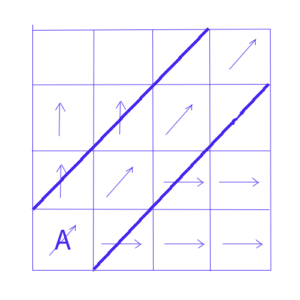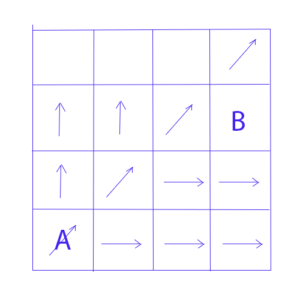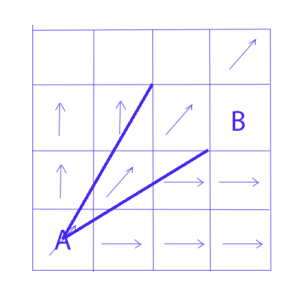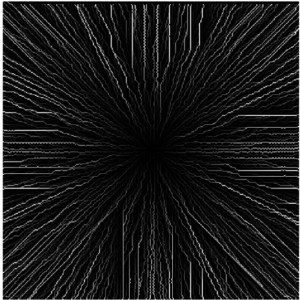### Hydrology with a GIS, for the Dummies (that we are): calculation of the flow (4)

In the last three articles, we have discussed an example of application for each of the main methods for calculating the flow of a watershed. Although they are quite self-explanatory, we must go through a little theory to complete the subject.
First of all, let’s see what happens with the most widespread method, mainly used for all ArcGis hydrological calculations, the D8 method.

D8 method: Analysis of the Problems

The D8 method allows the flow of each pixel to be directed towards a single receiving neighbouring cell. This method deals with flows that originate in one pixel (a two-dimensional surface, X and Y) as if it were a point source (dimensionless) and generates a downward flow line (with one dimension, the length) instead of a flow channel (with two dimensions, width and length). In short, we happily mix bath towels and tea towels. The single receiving neighbour cell, also imposes restrictions on the possible flow path configurations since the flow can be can be considered just in a cardinal direction (up, down, left, right) or diagonally.
The resulting errors in these boundaries are different for terrains where the flow is divergent (where the width of the flow path increases downhill), converge (the width of the flow path decreases downstream), or is parallel (the width of the flow path is constant along the slope).

The upstream pixels of a cell are called the Specific Contributing Surface (SCA) of that cell (the “watershed” of this cell).
The D8 method calculates the SCA accurately for a parallel flow (the width of the flow surface does not vary from upstream to downstream) only when the flow is in the x or y direction. When the flow forms an angle with respect to the orientation of the main grid, two types of errors occur:

 1-errors that affect the direction of the flow path and,

 2- SCA underestimation error for a given flow path.

The first source of error occurs when the flux forms an angle different from a multiple of 45 °. For example, if the flux forms an angle of 30 ° to the east (measured counter-clockwise), the steepest direction given by the D8 method will be to the NE, and each pixel will flow to its neighbour from NE.
This occurs by approximating the flow angle to 45 °; the modelled flow will be diverted from its true 15 ° path.
The second source of error results from the one-dimensional projection of the flow. Consider a parallel flow with an angle of 45 ° (or a multiple of 45 °).In the image, pixel A is the top of the slope.
The upstream flow surface will be calculated by a diagonal of pixels, contacting by their corners. For the pixel located at the top and on the right, it will receive, according to the calculation the rain fell on the three pixels located diagonally, up to the top.
Actually it should also include a part (half) of each pixel located to the left and right of these upstream pixels. The upstream flow area is therefore underestimated by a factor of 2 when using the D8 method.
When using D8, the calculated upstream surfaces for a parallel flow are accurate only for flows that occur precisely in a cardinal direction (0 °, 90 °, 180 °, or 270 °), are underestimated by a factor of two for flows that occur precisely in a diagonal direction (45 °, 135 °, 225 ° or 315 °), and are below  the correct value by a factor between 1 and 2 for the flows that occur between the cardinal directions and the diagonals.

For a divergent flow as the one we have in the semi-spherical DEM of the previous articles, results are quite different. Let’s first see how the D8 method works in this case.In the previous image, we consider that the pixel A is the top of the mountain, whether spherical or conical. Since we must get out of this pixel downwards, let’s suppose the method decides the flow is towards the NE pixel.
The arrows indicate the directions defined for the other pixels. Now consider the pixel B. None of the upstream pixels (West, South West and South contiguous pixels) are flowing to it. Therefore the method considers that there is no SCA, watershed, for this pixel. It is only the rain that falls on the pixel that will feed the downstream pixel.
In fact, its SCA is drawn on the following image:Actually, the pixel must  receive all the rain falling in the triangle formed by the top of the mountain and its two “lateral” corners.  Instead, the D8 method allocates the pixel an SCA = 0.

Let’s see what happens with the pixel to the left of B.In the case of an SCA substantially equal to that of the pixel B, we will obtain a computed SCA corresponding to the two pixels located towards the South West, thus SCA = 2. This is due to the fact that this pixel is on a direction that is a multiple of 45 ° from the top.

This phenomenon will replicate throughout the slope. We will have pixels at the bottom of the slope that, as pixel B, will have virtually no SCA, and others that will accumulate all along the slope. In the case of spherical or conical mountain flow, all the pixels at the bottom of the slope should have exactly the same SCA and the same cumulative flow.

In the case of  a convergent flow, as would be the case of a conical crater, we find the same type of problem but with discrepancies between the privileged directions (0,45,90, …) and the others, but  less marked.

In light of what has just been said, you can now better understand the result that we obtained in our other articles for the flow using the D8 method.The white “features”  in the privileged directions are the pixels that have recovered the SCA from their upstream pixels, the black areas at the edge of the image correspond to the pixels that happen to be as our pixel B of the example.

Rho8 method: Analysis of the Problems

The Rho8 method attempts to solve one of the D8 method problems, the deviation of the flow direction modelled towards a cardinal or diagonal direction. This is the result of the arbitrary structure of the grid where one can only choose one of these 8 directions.

The method used is based on chance and probabilities, which is never easy to explain. We will first try the real explanation:
The Rho8 method introduces a stochastic component in the D8 method, which makes it possible to better maintain the flow direction along a given slope and direction. As in the case of the D8 method, each pixel emerges in one of its eight neighbours. The choice of the reception pixel among the neighbours is performed stochastically. A probability p is assigned to one pixel, and a probability 1-p to a neighbouring pixel.

The Probability Attribution System and the purpose of this method are illustrated in the following example: Consider a flat surface with a 30 ° slope (measured counter clockwise from the East).  According to the D8 method, the flow will be towards the neighbouring pixel located at NE. The flow direction will, therefore, have a 15 ° difference with the true direction of flow. The Rho8 method assigns a probability p to the flow toward the NE neighbour and a probability of 1′-p to the flow toward the other possible neighbour, the east pixel.
As you go down the slope, some pixels will flow to their NE neighbour, and the rest will spill over to the east  neighbour. If the number of pixels that flow towards NE relative to the East neighbour respects the correct proportions (the expected proportion is p / (1 – p)), the resulting flux lines will have an overall direction of 30 °. The value of p shall be such that the expected value of the flow path direction is equal to the angle of the slope (“aspect”).

In more grotesque terms, if the conditions are maintained (length of the flow, etc.) the hazard causes the orientation to be equally modified in one direction as in the other and to get back, at the arrival, to the original orientation.

Although this method provides (in mathematical expectation) the appropriate flow path direction, all other problems identified for method D8 persist. But in addition, the Rho8 method introduces its own problems: chance does not guarantee reproducible results, and in locations with parallel flows, the adjacent flow paths are not parallel, but randomly deviated, which causes, often, a convergence of flows that should be parallel. This happens in particular on flat surface portions, where the flux paths should remain parallel and where, instead, we find pixels with strong accumulations.

Once two flow paths have merged because of their random deviation, there is no mechanism that can cause them to diverge again. As you move down the slope, the errors increase as the flow becomes more and more concentrated.

In the end, some pixels will have overestimated SCA values, while others that have been neglected by the randomly deviated flow streams will depict underestimated values.MFD method: Analysis of the Problems

The multi-directional methods attempt to solve the major limitation of the D8 method, i.e. the one-dimensional representation of the flow, by distributing fluxes from one pixel to all the neighbouring pixels that are located below it. The rule used is to distribute the respective flows to those neighbours which change, according to the method used.

We will not discuss the details of the  MFD method which is based on the directional slope of the pixels, but, let’s just say that in any possible scenario, each pixel will flow to three or four other pixels depending on the slope.

Besides, it will receive the flow of three or four neighbouring upstream pixels, and this flow will not be complete (as in the D8 method), but partial.
On an inclined plane, whether conical or circular plan, if we draw the upstream zone of each pixel, we can observe that it constitutes an inverted triangle, with the vertex situated on the pixel considered and the base on the upstream boundary of the plan.

The SCA value calculated for a pixel (the contributory surface or upstream basin) is correct, if and only if, it is far enough from the edges of the area covered by the DTM. If this is the case, the triangular zone is included in the DTM, otherwise the triangular region is incomplete and the SCA is underestimated.

A parameter of the method (the convergence) makes it possible to offset to a certain degree this problem. For a mountain in the shape of a circular cone, a value of 1.1 has given very good results. The worry is that this “calibration” is based on the geometric symmetry of the surface and the measurement will be compromised since the natural surface of the ground deviates from the symmetrical geometry of the surfaces used for the calibration of the method.

Conclusion (if there is one …)

We will conclude here this series of articles, not because the subject can be considered closed, but on the contrary, because the subject can be considered definitely open.

The purpose of these articles is not to provide the answers, but to ask the questions. Once asked, these questions, we will approach the hydrological modelling of a field more humbly. And, to warn everyone to continue his journey trying to answer the new questions that will, undoubtedly arise.